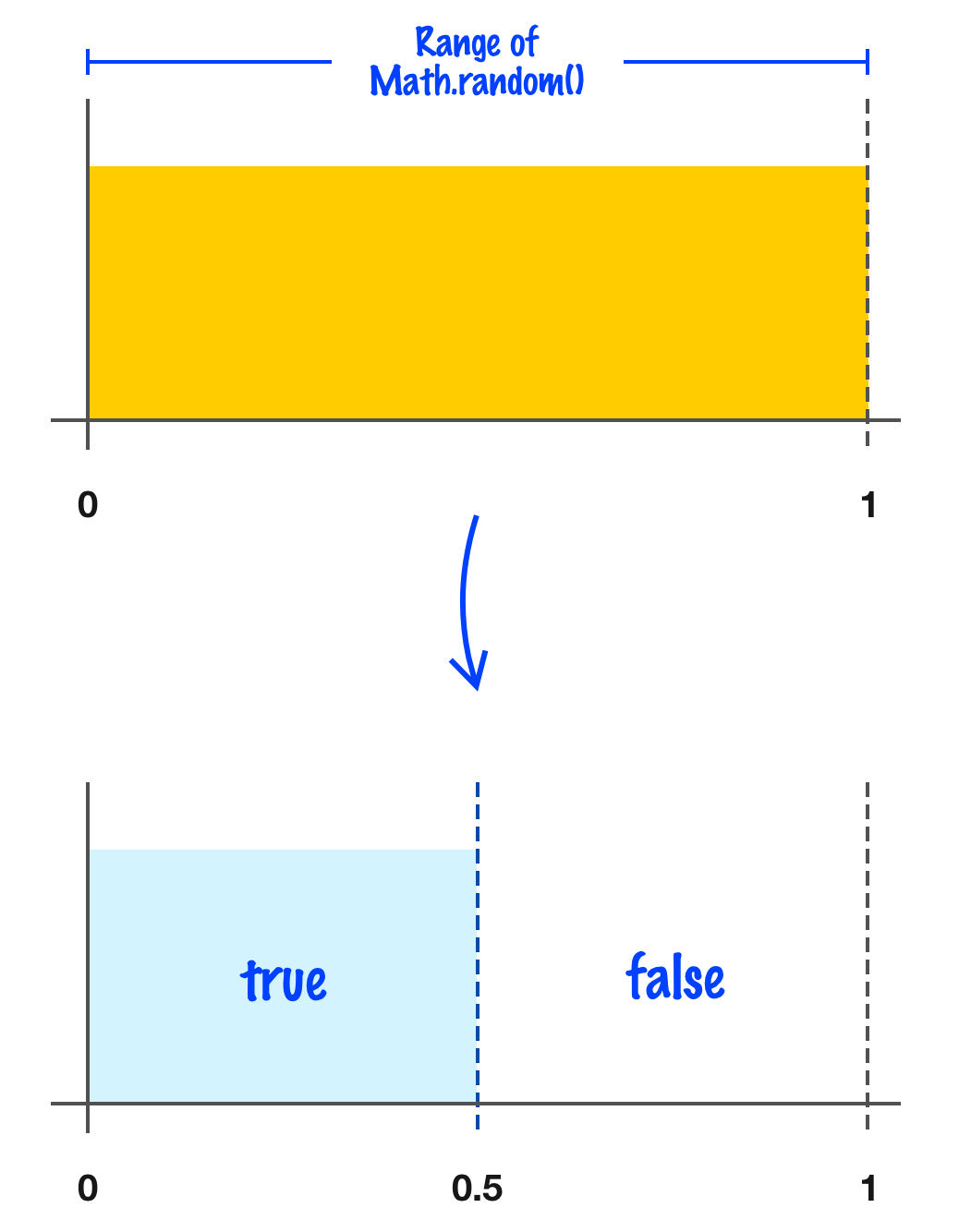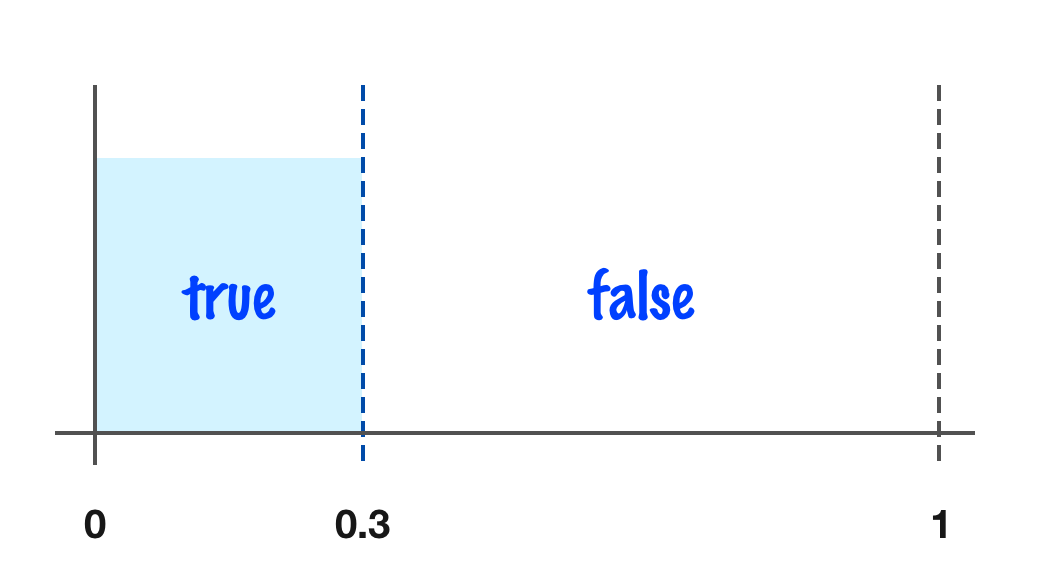﻿ Generating a Random True or False Boolean Value

Color

Background

Done

# Generating a Random True or False Boolean Value

by kirupa   |   filed under JS Tips and Tricks

When we think of generating a random number, we typically think of getting a value that falls between a wide range of values. What if we adjusted our thinking a bit and scoped the range of values that get randomly generated down to just two values instead? Why would we want to do this? Watch the video or read the full article:

For many situations, we'll want our code to make a choice between one of two outcomes. This would be the digital equivalent of making a decision by tossing a coin:In a coin toss, the output would would either be heads or tails. Assuming the coin is fair and no tricks are involved, it's safe to assume that one has an equal chance of seeing a value of heads or tails with each throw. The randomly pick one of two values idea behind the coin toss is core to many computer applications. Some examples include behaviors in video games, calculations in cryptography, steps in popular algorithms, inputs to artificial intelligence models, and more. In this article, we'll look at one approach for generating a value that randomly flips between one of two values. We'll look at how to randomly generate a true or false.

Onwards!

## Looking at the Code

Jumping head first into all of this, let's look at the code for generating a random boolean value of true or false:

``````function getRandomBoolean() {
return Math.random() < 0.5;
}

console.log(getRandomBoolean()); // true or false``````

When we run this code and call getRandomBoolean, we will get a value of either true or false. If you keep calling this function long enough, you'll find that both true and false appear with a certain level of consistency. That's pretty cool!

Going on level deeper, what makes our code work is the Math.random() < 0.5 expression. From the Random Numbers in JS article, we know that Math.random() returns a number whose range is between 0 and less than 1. In other words, the upper range is very VERY close to 1, but it never quite reaches it. Using that detail, we can visualize what we are doing as follows:Dividing our range into two equal halves, we can say that any value returned by Math.random() that is less than .5 is true. That is exactly what our code is doing. To repeat something we saw at the beginning, what our code simulates is the digital equivalent of a fair coin flip.

#### Note: Creating an Unfair True/False Coin Flip

The same approach we saw above for allowing our code to give us an equal chance of seeing true and false can be used to create an unfair situation as well. By adjusting the value we compare Math.random() against, we can adjust the frequency that we see true and false in. For example, let's say we adjust our expression to be Math.random() < 0.3. This can be visualized as follows:What this means is that our code will return a value of true only 30% of the time. By simply playing with some numbers, we have a lot of power in skewing the balance of true and false results in unfair ways.

## Conclusion

When it comes to random numbers, we just have one job. That job is to learn as much as we can about how Math.random() operates and any quirks we can rely on. What we saw in this article is how to generate true or false randomly by relying on the exact range Math.random() will return a value in. To verify that our approach does give us a fair distribution, we can do a really quick test that is far less involved than the Random Number Frequency Visualizer:

``````function getRandomBoolean() {
return Math.random() < 0.5;
}

let trueValue = 0;
let falseValue = 0;

for (let i = 0; i < 100000; i++) {
if (getRandomBoolean()) {
trueValue++;
} else {
falseValue++;
}
}

console.log(`TRUE count is \${trueValue}, and FALSE count is \${falseValue}`);``````

If you run the above code, you will see a nearly 50/50 split between true and false being generated. If you alter the value we compare Math.random() against from 0.5 to something else (like 0.3 as highlighted in the Note), you'll see that balance reflected as well.

Just a final word before we wrap up. If you have a question and/or want to be part of a friendly, collaborative community of over 220k other developers like yourself, post on the forums for a quick response!